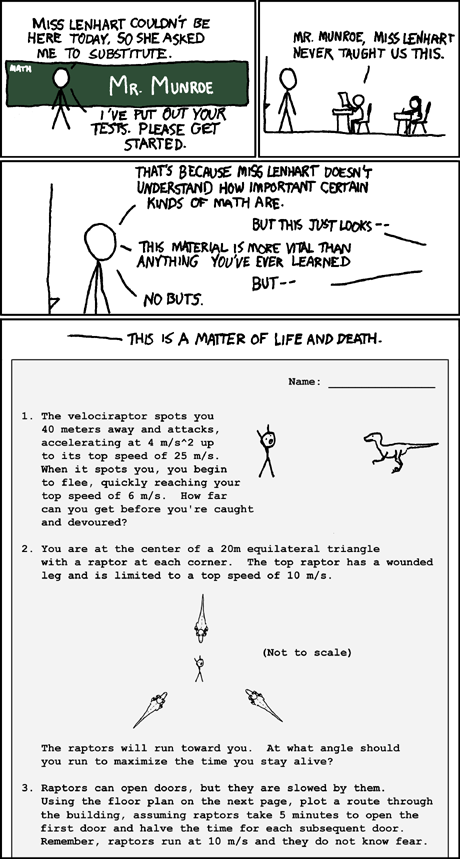# Practical level 5 Maths

## Course Overview

Code:
LA Code:
Levels:
5
Learning Area:

Maths with Matt - what could be better?

This course will cover the Level 5 Maths curriculum with a more practical/ context-based approach. We will NOT be doing any NCEA internal assessments, but you should finish the year prepared for NCEA level 1 NEXT year. We will cover a smaller quantity of content, at a slower pace than Alisha and Duncan's level 5 classes.

In maths students will explore relationships in quantities, space and data and learn to express these relationships in ways that help them to make sense of the world around them.
Learning maths should help students to become knowledgeable citizens in an information and data driven time.
The fundamental learning outcomes in maths are to develop -
The ability to think creatively, critically and logically
The ability to structure and organize
The ability to process information
The ability to interpret and critically evaluate statistical information in a variety of contexts
The ability to solve problems that help them to investigate and understand the worldTERM 1

Block 1.1 NUMBER

Use prime numbers, common factors, multiples powers and square roots.
Understand operations on fractions, decimals, percentages and integers
Use rates and ratios
Know commonly used fraction, decimal and percentage conversions
Know and apply standard form, significant figures, rounding and decimal place value

Block 1.2 MEASUREMENT

Select and use appropriate metric units for length, area, volume and capacity, weight, temperature, angle and time
Convert between metric units using decimals
Deduce and use formulae to find the perimeters and areas of polygons and the volumes of prisms

Find the perimeters and areas of circles and composite shapes and the volumes of prisms including cylinders

TERM 2

Block 2.1/2.2 ALGEBRA / GRAPHS

Form and solve linear and simple quadratic graphs
Generalise the properties of operations with fractional numbers and integers
Relate tables, graphs and equations to linear and simple quadratic relationships found in number and spatial patterns

TERM 3

Block 3.1 STATISTICS

Plan and conduct surveys and experiments using the statistical enquiry cycle -
Determining appropriate variables and measures
Considering sources of variation
Gathering and cleaning data
Using multiple displays and re-categorising data to find patterns, variations, relationships and trends in multivariate data sets
Comparing sample distributions visually, using measures of centre, spread and proportion
Presenting a report of findings
Evaluate statistical investigations or probability activities undertaken by others, including data collection methods, choice of measures and validity of findings.

Block 3.2 GEOMETRY

Deduce the angle properties of intersecting and parallel lines and the angle properties of of polygons and apply these properties
Create accurate nets for simple polyhedral and connect 3-D solids with different 2-D representations
Construct and describe simple loci
Interpret points and lines on co-ordinate planes, including scales and bearings on maps
Apply trigonometric ratios and Pythagoras’ theorem in two dimensions

TERM 4

Block 4.1 PROBABILITY

Compare and describe the variation between theoretical and experimental distributions in situations that involve elements of chance
Calculate probabilities, using fractions, percentages and ratio

## Courses offered per block.

TitleLA CodeBlockYear